# Zernike polynomials

(diff) ← Older revision | Latest revision (diff) | Newer revision → (diff)

Polynomials (cf. also Polynomial) constructed by F. Zernike [a5] and by Zernike and H. Brinkman [a6] for the purpose of approximating certain functions, such as the aberration function of geometrical optics, on the disc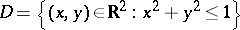. The underlying premise is that errors in circular optical elements can be quantified by mean-square deviation per unit area. Given a functiononand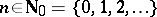, the problem of finding a polynomialof degreewhich minimizes the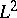-norm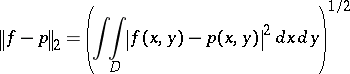is solved by means of orthogonal polynomials (cf. Orthogonal polynomials). This means that for each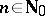there is an orthogonal basis for the spaceof polynomials of degree, which are orthogonal to each polynomial of lower degree (orthogonality is with respect to the inner product). The dimension of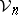is. In the case of the disc there are at least two useful approaches to constructing orthogonal polynomials, based on the Cartesian or on the polar coordinate system. The Zernike polynomials are associated with the polar coordinate system (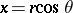,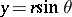) and with complex coordinates (,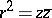). Forand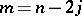with, the Zernike circle polynomial iswhere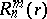is a polynomial of degreein, of the same parity as. This family has been generalized to "disc polynomials" , associated with the weight function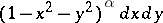with arbitrary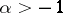(see [a3]). The formulas will be stated for the general case since they are no more complicated than for the Zernike polynomials (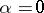). A convenient indexing is obtained from setting,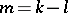for arbitrary. Then (using the Pochhammer symboland the hypergeometric function), define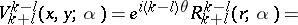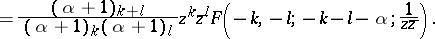The normalization of the polynomials comes from the equation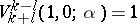. The orthogonality relations are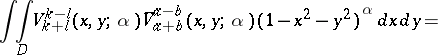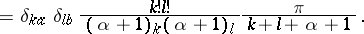The polynomials can be expressed in terms of Jacobi polynomials: for,and satisfy a Rodrigues formula:The orthogonal polynomials of degree(that is,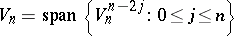) satisfy a differential equation: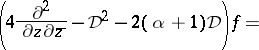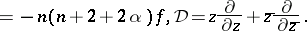There is an important integral transform used in the diffraction theory of aberrations (see [a1], Chap. 9): Let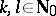,, and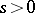, thenwheredenotes the Bessel function of index(cf. Bessel functions). The coefficients of the orthogonal expansion of an aberration function in terms of the Zernike polynomials are related to the so-called primary aberrations (such as astigmatism, coma, distortion), see [a1], Chap. 5. The disc polynomials forappear as spherical functions on the homogeneous spaces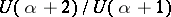(wheredenotes the unitary group, see [a2], Vol. 2, Sec. 11.5, pp. 359–363). The Zernike polynomials are key tools in two-dimensional tomography; see [a4].